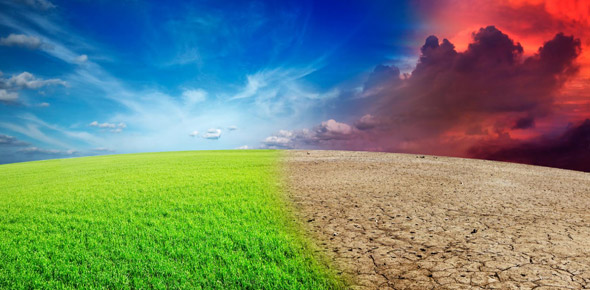# Matter In Our Surroundings

15 Questions | Total Attempts: 4015SettingsThis somewhat geographical-somewhat chemical quiz will put your knowledge to the test on assorted topics such as the Kelvin scale, dry ice, inter-particle forces, the melting point of a substance and the evaporation of liquids.

Related Topics
• 1.
Which one of the following sets of phenomena would increase on raising the temperature?
• A.

Diffusion ,evaporation, compression of gases

• B.

Evaporation, compression of gases and solubility

• C.

Evaporation , diffusion , expansion of gases

• D.

Evaporation, solubility diffusion compression of gases

• 2.
• A.

Low temperature , low pressure

• B.

High temperature , low pressure

• C.

Low temperature , high pressure

• D.

High temperature , high pressure

• 3.
On Kelvin scale 0OC is equal to –
• A.

273 K

• B.

– 273 K

• C.

0 K

• D.

100 K

• 4.
The melting point of a substance is defined as the constant temperature at 1 Atmospheric pressure when
• A.

Both the solid & liquid exist together

• B.

Only liquid is present

• C.

The solid is completely changed into liquid

• D.

The solid starts melting

• 5.
Which of the following factors are responsible for the change of state of solid CO2 into Vapours?
• A.

Increase in temperature

• B.

Decrees In pressure

• C.

Increase in pressure

• D.

Both decrees in pressure and increase in temperature

• 6.
Dry ice means –
• A.

Solid

• B.

Solid water

• C.

Solid

• D.

Solid CO

• 7.
When we add sugar in water, particles of sugar disappear because they -
• A.

Are very small

• B.

Get into the spaces between water particles

• C.

Are moving

• D.

All above

• 8.
Find the incorrect statement
• A.

Cooling is caused during boiling

• B.

Low boiling liquids evaporate faster than high boiling liquids

• C.

Evaporation is a surface phenomena but boiling is not

• D.

The standard room temperature is 298K

• 9.
The evaporation of a liquid occur only at
• A.

All temperatures

• B.

A fixed temperature

• C.

Temperature less than 0°C

• D.

Temperature more than 100°C

• 10.
The interparticle forces are the strongest in
• A.

Ammonia

• B.

Carbon dioxide

• C.

Sodium Bromide

• D.

Ethyl alcohol

• 11.
During summer water kept in an earthen pot becomes cool because of the phenomenon of
• A.

Diffusion

• B.

Transpiration

• C.

Osmosis

• D.

Evaporation

• 12.
Select the correct order of substances in increasing order of attraction between the particles:
• A.

Water < Sugar < Oxygen

• B.

Sugar < Oxygen < Water

• C.

Sugar < Water < Oxygen

• D.

Oxygen < Water < Sugar

• 13.
Name the state of matter that 'has minimum inter particle attraction’
• A.

Solid

• B.

Gas

• C.

Liquid

• D.

All of these

• 14.
The melting point of a substance is defined as the constant temperature at 1 Atmospheric pressure when
• A.

Both the solid & liquid exist together

• B.

Only liquid is present

• C.

The solid is completely changed into liquid

• D.

The solid starts melting

• 15.
• A.

Conversion of solid into vapors without passing through the liquid state is called as vaporization

• B.

Conversion of vapors into solids without passing through the liquid state is called sublimation

• C.

Conversion of vapors into solids without passing through the liquid state is called freezing

• D.

Conversion of solid into liquid is called sublimation